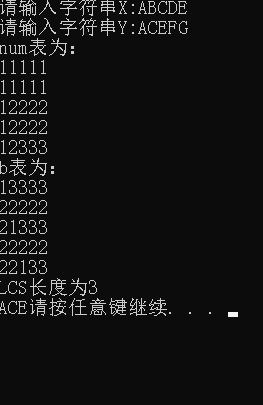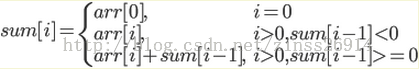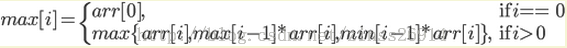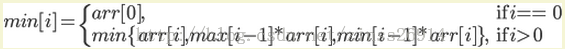• 最长公共子序列问题C语言#include#include#includeint num={0};int b={0};int lcsLength(char *x,char *y,int xLen,int yLen);void LCS(int i,int j,char *x);int lcsLength(char *x,char *y,int ...

最长公共子序列问题C语言
#include#include#includeint num={0};
int b={0};
int lcsLength(char *x,char *y,int xLen,int yLen);
void LCS(int i,int j,char *x);
int lcsLength(char *x,char *y,int xLen,int yLen){
int m=xLen-1;
int n=yLen-1;
for(int i=0;i<=m;i++){
for(int j=0;j<=n;j++){
if(x[i]==y[j]){
num[i][j]=num[i-1][j-1]+1;
b[i][j]=1;
}else if(num[i-1][j]>=num[i][j-1]){
num[i][j]=num[i-1][j];
b[i][j]=2;
}else{
num[i][j]=num[i][j-1];
b[i][j]=3;
}
}
}
测试正确性的矩阵图
printf("num表为：\n");
for(int i=0;i<=m;i++){
for(int j=0;j<=n;j++){
printf("%d",num[i][j]);
}
printf("\n");
}
printf("b表为：\n");
for(int i=0;i<=m;i++){
for(int j=0;j<=n;j++){
printf("%d",b[i][j]);
}
printf("\n");
}
/
return num[m][n];
}
void LCS(int i,int j,char *x){
if(i<0||j<0) return ;
if(b[i][j]==1){
LCS(i-1,j-1,x);
printf("%c",x[i]);
}else if(b[i][j]==2){
LCS(i-1,j,x);
}
else LCS(i,j-1,x);
}
void main(){
char x,y;
printf(“请输入字符串X:”);
scanf("%s",x);
while(strlen(x) > 50)
{
printf(“您输入的字符串超过最大长度,请重新输入!”);
scanf("%s",x);
}
printf(“请输入字符串Y:”);
scanf("%s",y);
while(strlen(y) > 50)
{
printf(“您输入的字符串超过最大长度,请重新输入!”);
scanf("%s",y);
}
int xLen=strlen(x);
int yLen=strlen(y);
printf(“LCS长度为%d\n”,lcsLength(x,y,xLen,yLen));
LCS(xLen-1,yLen-1,x);
system("pause");
}展开全文• 题目描述：给一个浮点数序列，取最大乘积连续子串的值，...提醒：此最大乘积连续子串与最大乘积子序列不同，请勿混淆，前者子串要求连续，后者子序列不要求连续。也就是说：最长公共子串(Longest CommonSubstring...

题目描述：给一个浮点数序列，取最大乘积连续子串的值，例如 -2.5，4，0，3，0.5，8，-1，则取出的最大乘积连续子串为3，0.5，8。也就是说，上述数组中，3 0.5 8这3个数的乘积3*0.5*8=12是最大的，而且是连续的。
提醒：此最大乘积连续子串与最大乘积子序列不同，请勿混淆，前者子串要求连续，后者子序列不要求连续。也就是说：最长公共子串(Longest CommonSubstring)和最长公共子序列(LongestCommon Subsequence，LCS)的区别：
子串(Substring)是串的一个连续的部分，子序列(Subsequence)则是从不改变序列的顺序，而从序列中去掉任意的元素而获得的新序列；
更简略地说，前者(子串)的字符的位置必须连续，后者(子序列LCS)则不必。比如字符串“ acdfg ”同“ akdfc ”的最长公共子串为“ df ”，而它们的最长公共子序列LCS是“ adf ”，LCS可以使用动态规划法解决。
解答：
解法一、穷举，所有的计算组合：
或许，读者初看此题，自然会想到最大乘积子序列问题类似于最大子数组和问题：http://blog.csdn.net/v_JULY_v/article/details/6444021，可能立马会想到用最简单粗暴的方式：两个for循环直接轮询。
解法二、虽说类似于最大子数组和问题，但实际上具体处理起来诸多不同。为什么呢，因为乘积子序列中有正有负也还可能有0。我们可以把问题简化成这样：数组中找一个子序列，使得它的乘积最大；同时找一个子序列，使得它的乘积最小(负数的情况)。因为虽然我们只要一个最大积，但由于负数的存在，我们同时找这两个乘积做起来反而方便。也就是说，不但记录最大乘积，也要记录最小乘积。So，我们让maxCurrent表示当前最大乘积的candidate，minCurrent反之，表示当前最小乘积的candidate，而maxProduct则记录到目前为止所有最大乘积candidates的最大值。(以上用candidate这个词是因为只是可能成为新一轮的最大/最小乘积)由于空集的乘积定义为1，在搜索数组前，maxCurrent，minCurrent，maxProduct都赋为1。
假设在任何时刻你已经有了maxCurrent和minCurrent这两个最大/最小乘积的candidates，新读入数组的元素x(i)后，新的最大乘积candidate只可能是maxCurrent或者minCurrent与x(i)的乘积中的较大者，如果x(i)<0导致maxCurrent
解法三、本题除了上述类似最大子数组和的解法，也可以直接用动态规划求解(其实，上述的解法一本质上也是动态规划，只是解题所表现出来的具体形式与接下来的解法二不同罢了。这个不同就在于下面的解法二会写出动态规划问题中经典常见的DP方程，而解法一是直接求解)。具体解法如下：
假设数组为a[]，直接利用动归来求解，考虑到可能存在负数的情况，我们用Max来表示以a结尾的最大连续子串的乘积值，用Min表示以a结尾的最小的子串的乘积值，那么状态转移方程为：
Max=max{a, Max[i-1]*a, Min[i-1]*a};
Min=min{a, Max[i-1]*a, Min[i-1]*a};
初始状态为Max=Min=a。
下面来看一道具体的ACM题目
题目
题目描述：
给定一个浮点数序列(可能有正数、0和负数)，求出一个最大的连续子序列乘积。
输入：
输入可能包含多个测试样例。
每个测试样例的第一行仅包含正整数 n(n<=100000)，表示浮点数序列的个数。
第二行输入n个浮点数用空格分隔。
输入数据保证所有数字乘积在双精度浮点数表示的范围内。
输出：
对应每个测试案例，输出序列中最大的连续子序列乘积，若乘积为浮点数请保留2位小数，如果最大乘积为负数，输出-1。
样例输入：
7
-2.5 4 0 3 0.5 8 -1
5
-3.2 5 -1.6 1 2.5
5
-1.1 2.2 -1.1 3.3 -1.1
样例输出：
12
64
8.78
思路最大连续子序列乘积和最大连续子序列和不同，这里先回忆一下最大连续子序列和的最优解结构：
最大连续子序列和
我们用sum[i]来表示以arr[i]结尾的最大连续子序列和，则状态转移方程为：最大连续子序列乘积
考虑存在负数的情况(ps：负负会得正)，因此我们用两个辅助数组，max[i]和min[i],max[i]来表示以arr[i]结尾的最大连续子序列乘积，min[i]来表示以arr[i]结尾的最小连续子序列乘积，因此状态转移方程为：and有了状态转移方程，dp代码就很容易实现了，看到这里还不理解的同学，我建议你多花点时间用在算法学习上吧！
AC代码
#include
#include
double maxNumInThree(double a, double b, double c)
{
double max;
max = (a > b) ? a : b;
max = (max > c) ? max : c;
return max;
}
double minNumInThree(double a, double b, double c)
{
double min;
min = (a < b) ? a : b;
min = (min < c) ? min : c;
return min;
}
int main(void)
{
int i, n;
double *arr, *max, *min, res;
while (scanf("%d", &n) != EOF) {
arr = (double *)malloc(sizeof(double) * n);
max = (double *)malloc(sizeof(double) * n);
min = (double *)malloc(sizeof(double) * n);
for (i = 0; i < n; i ++)
scanf("%lf", arr + i);
// 动态规划求最大连续子序列乘积
max = min = res = arr;
for (i = 1; i < n; i ++) {
max[i] = maxNumInThree(arr[i], max[i - 1] * arr[i], min[i - 1] * arr[i]);
min[i] = minNumInThree(arr[i], max[i - 1] * arr[i], min[i - 1] * arr[i]);
if (max[i] > res)
res = max[i];
}
if (res >= 0) {
// 判断是否为浮点数
if ((res - (int)res) == 0)
printf("%d\n", (int)res);
else
printf("%.2lf\n", res);
} else {
printf("-1\n");
}
free(arr);
}
return 0;
}
/**************************************************************
Problem: 1501
User: wangzhengyi
Language: C
Result: Accepted
Time:110 ms
Memory:4964 kb
****************************************************************/

展开全文• /*取c[i-1][j]和c[i][j-1]的最大值,并记录c[i][j]是通过哪一个子问题的值求得的，以决定搜索的方向*/   int  Max( int  m, int  n, int  i, int  j)  {    if (m > n)   {   b[i][j] = -1;    ...
#include<stdio.h>  #include<string.h>  int c;   //用c[i][j]记录X[i]与Y[j] 的LCS 的长度  int b;   //b[i][j]记录c[i][j]是通过哪一个子问题的值求得的，以决定搜索的方向  char f;    /*-----------------------分割线--------------------------------*/    /*取c[i-1][j]和c[i][j-1]的最大值,并记录c[i][j]是通过哪一个子问题的值求得的，以决定搜索的方向*/  int Max(int m,int n,int i,int j)  {      if(m > n)      {          b[i][j] = -1;          return m;      }      else      {          b[i][j] = 1;          return n;      }  }  /*-----------------------分割线--------------------------------*/  /*递归打印LCS的元素内容*/  void print(int i,int j,int s,char x[],char y[])  {      if(b[i][j] == 0)      {          f[s-1] = x[i-1];          i--;j--;s--;          print(i,j,s,x,y);      }      else if(b[i][j] == -1)      {          i--;          print(i,j,s,x,y);      }      else if(b[i][j] == 1)      {          j--;          print(i,j,s,x,y);      }  }  /*-----------------------分割线--------------------------------*/  int LCS(char x[],char y[])  {      int i,j;      int x_len,y_len;      x_len = strlen(x);      y_len = strlen(y);      printf("   ");      for(i = 0;i < y_len;i++)      {          printf("%c  ",y[i]);      }      printf("\n");      for(i = 1;i <= x_len;i++)      {          printf("%c  ",x[i-1]);          for(j = 1;j <= y_len;j++)          {              if(x[i-1] == y[j-1])              {                  c[i][j] = c[i-1][j-1] +1;                  b[i][j] = 0;                  printf("%d  ",c[i][j]);              }              else              {                  c[i][j] = Max(c[i-1][j],c[i][j-1],i,j);                  printf("%d  ",c[i][j]);              }          }          printf("\n");      }      /*-------------------------分割线---------------------------------------*/      //打印X和Y的LCS      printf("X和Y的LCS是:");      print(x_len,y_len,c[x_len][y_len],x,y);      printf("%s",f);      printf("\n");      return c[x_len][y_len];  }    /*------------------------------分割线----------------------------------------*/  void main()  {      char X,Y;      int i,j,s;      printf("请输入字符串X:");      scanf("%s",X);      while(strlen(X) > 200)      {          printf("您输入的字符串超过最大长度,请重新输入!");          scanf("%s",X);      }      printf("请输入字符串Y:");      scanf("%s",Y);      while(strlen(Y) > 200)      {          printf("您输入的字符串超过最大长度,请重新输入!");          scanf("%s",Y);      }      s = LCS(X,Y);      printf("X和Y的LCS: %d \n",s);  }
展开全文• 最长公共子序列 & 最长公共子串的区别：找两个字符串的最长公共子串，这个子串要求在原字符串中是连续的。而最长公共子序列则并不要求连续。leetcode 1143题 最长公共子序列！！！字符可以不连续给定两个字符串 ...

最长公共子序列 & 最长公共子串的区别：
找两个字符串的最长公共子串，这个子串要求在原字符串中是连续的。而最长公共子序列则并不要求连续。
leetcode 1143题 最长公共子序列
！！！字符可以不连续
给定两个字符串 text1 和 text2，返回这两个字符串的最长公共子序列。
一个字符串的 子序列 是指这样一个新的字符串：它是由原字符串在不改变字符的相对顺序的情况下删除某些字符(也可以不删除任何字符)后组成的新字符串。
例如，“ace” 是 “abcde” 的子序列，但 “aec” 不是 “abcde” 的子序列。两个字符串的「公共子序列」是这两个字符串所共同拥有的子序列。
若这两个字符串没有公共子序列，则返回 0。
示例 1:
输入：text1 = “abcde”, text2 = “ace”
输出：3
解释：最长公共子序列是 “ace”，它的长度为 3。
示例 2:
输入：text1 = “abc”, text2 = “abc”
输出：3
解释：最长公共子序列是 “abc”，它的长度为 3。
示例 3:
输入：text1 = “abc”, text2 = “def”
输出：0
解释：两个字符串没有公共子序列，返回 0。
提示:
1 <= text1.length <= 1000
1 <= text2.length <= 1000
输入的字符串只含有小写英文字符。
# leetcode submit region begin(Prohibit modification and deletion)
class Solution(object):
def longestCommonSubsequence(self, text1, text2):
"""
:type text1: str
:type text2: str
:rtype: int
"""
t1,t2 = len(text1),len(text2)
dp = [[0 for j in range(t2+1)] for i in range(t1+1)]
# 0行和0列都是字符串和空字符比较，为0
for i in range(1,t1+1):
for j in range(1,t2+1):
# 如果匹配 则移除i，j对应的字符，结果+1
# 然后再去找之前的最大值
if text1[i-1]==text2[j-1]:
dp[i][j] = 1 + dp[i-1][j-1]
else:
# 如果不匹配，则为0， 去找左边或者右边最大值
dp[i][j] = max(dp[i-1][j],dp[i][j-1])
#print(dp[i][:])
return(dp[-1][-1])
# leetcode submit region end(Prohibit modification and deletion)
字符串a,b中的最长公共子串
题目描述
查找两个字符串a,b中的最长公共子串。若有多个，输出在较短串中最先出现的那个。
输入描述:
输入两个字符串
输出描述:
返回重复出现的字符
while True:
try:
str1,str2 = input(),input()
# 短的为text1 长的为text2
text1, text2 = (str1, str2) if len(str1) < len(str2) else (str2, str1)
t1,t2 = len(text1),len(text2)
dp = [[0 for j in range(t2+1)] for i in range(t1+1)]
maxlen = 0
for i in range(1,t1+1):
for j in range(1,t2+1):
# 如果匹配 则移除i，j对应的字符，去找之前的最大值
if text1[i-1]==text2[j-1]:
dp[i][j] = 1 + dp[i-1][j-1]
if dp[i][j]>maxlen:
# 记录最长公共子串的长度
maxlen = dp[i][j]
start = i - maxlen
# 如果不匹配，则为0
print(text1[start:start+maxlen])
except:
break
最长上升子序列
给定一个无序的整数数组，找到其中最长上升子序列的长度。
示例:
输入: [10,9,2,5,3,7,101,18]
输出: 4
解释: 最长的上升子序列是 [2,3,7,101]，它的长度是 4。
说明: 可能会有多种最长上升子序列的组合，你只需要输出对应的长度即可。
# 进阶: 你能将算法的时间复杂度降低到 O(n log n) 吗?
# Related Topics 二分查找 动态规划
# leetcode submit region begin(Prohibit modification and deletion)
class Solution(object):
def lengthOfLIS(self, nums):
"""
:type nums: List[int]
:rtype: int
"""
if len(nums) == 0:
return 0
n = len(nums)
lengths = [1 for _ in range(n)]
for i in range(1,n):
for j in range(i):
# 坐标锁定在i， 然后遍历j(从0->i-1)
if nums[j] < nums[i]:
lengths[i] = max(lengths[j] + 1,lengths[i])
#print(lengths)
return(max(lengths))
# leetcode submit region end(Prohibit modification and deletion)

展开全文• 最长公共子序列问题C语言 #include<stdio.h> #include<stdlib.h> #include<string.h> int num={0}; int b={0}; int lcsLength(char *x,char *y,int xLen,int yLen); void LCS(int...算法
• 最长公共子序列.（C语言编写） 算法最长公共子序列.（C语言编写） 算法最长公共子序列.（C语言编写） 算法
• 《算法导论》中动态规划的第三个问题是最大子序列 这里的最大子序列的定义是：找出一个序列S3，S3在S1和S2中都出现，且出现的顺序相同，现在找出最长的一个S3。 对于序列A=“ABCBDAB”，B=“BDCABA” 他的一个最大子...算法 动态规划 数据结构
• （1）将输入的两个字符串分别存在数组a[]和b[]中，同时设置两个临时数组t1[][]和t2[][]，第一个t1[i][j]表示a[]数组前i-1个字符串和b[]数组前j-1个字符串的最大公共子序列；第二个t2[i][j]表示a[]数组前i-1个字符串...算法
• 最长公共子序列 最长公共子序列的求解，如下图所示： 可以看到，最长公共子序列的依赖关系如上图所示，[i,j]的值取决于{[i-1,j-1],[i,j-1],[i-1,j]} 实现方法如下： LCS_len.h #include #include #include #define ...
• 一、求最长公共子序列 1、问题描述： 从一个给定的串中删去（不一定连续地删去）0个或0个以上的字符，剩下地字符按原来顺序组成的串。例如：“ ”，“a”，“xb”，“aaa”，“bbb”，“xabb”，“xaaabbb”都是串...动态规划
• 给定两个字符串text1 和text2，返回这两个字符串的最长公共子序列。 一个字符串的子序列是指这样一个新的字符串：它是由原字符串在不改变字符的相对顺序的情况下删除某些字符（也可以不删除任何字符）后组成的新...
• #include <stdio.h> #include <string.h> #include <math.h> int Max(int a,int b) { if(a>b) return a; else return b;... if(strlen(a)==0 || strlen(b)==0 |...算法
• 最近看了很多关于LCS(Longest common subsequence problem,最长公共子序列)的文章，大部分问题都只是求出最大公共子序列的长度，或者打印处其中的任意一个最大子序列即可，但是如何快速的打印出所有的最大长度子...
• 要求在text中找出以同样的顺序连续出现在query中的最长连续字母序列的长度。例如， query为“acbac”，text为“acaccbabb”，那么text中的“cba”为最长的连续出现在query中的字母序列，因此，返回结果应该为其长度3...string
• 这段时间，一直再看《算法导论》，现在看到了动态规划这部分，其中的一个 最大公共序列的问题，挺有意思，用到了动态规划的思想，具体的原理可以参考《算法导论》的第15章，page208.  于是就用C语言实现了...c C 算法
• 一.前言 如上 ...//求最长公共子序列的长度 //#include<string.h> int main(){ int max(int a,int b); int i;//循环变量 int j;//循环变量 int a; char s2;//B char s1;//A算法 动态规划
• //最大公共子序列的问题 #include #include #define MAXSIZE 200 int c; int b; char f; void print(int i,int j,int s,char x[],char y[]); int max(int m,int n,int i,int j)//c[i][j-1]...算法基础
• 分析并掌握“最长公共子序列” 问题的动态规划算法求解方法； 最长公共子序列问题：若给定序列X={x1,x2,…,xm}，则另一序列Z={z1,z2,…,zk}，是X的子序列是指存在一个严格递增下标序列{i1,i2,…,ik}使得对于所有j=1...
• 【问题】 求两字符序列的最长公共字符子序列 问题描述：字符序列的子序列是指从给定字符序列中随意地（不一定连续）去掉若干个字符（可能一个也不去掉）后所形成的字符序列。令给定的字符序列X=“x0，x1，…，xm-1...动态规划
• 最近看了很多关于LCS(Longest common subsequence problem,最长公共子序列)的文章，大部分问题都只是求出最大公共子序列的长度，或者打印处其中的任意一个最大子序列即可，但是如何快速的打印出所有的最大长度子序列...动态规划 dp 算法
•  *递归小程序_求两个串的最大公共子序列的长度  * 1、参数要一定变化  * 2、每次都要降低规模  */ public class PublicLength {  public static void main(String[] args) {  int k = f("fabckdE","xbacdE"); ...递归
• 题目描述：给一个浮点数序列，取最大乘积连续子串的值，...提醒：此最大乘积连续子串与最大乘积子序列不同，请勿混淆，前者子串要求连续，后者子序列不要求连续。也就是说：最长公共子串(Longest CommonSubstring......

# 最大公共子序列c语言c语言 订阅